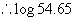## How to Use Logarithms (cont.)

 Examples: Although a four-place log table is used here, for purposes where accuracy to 3 significant figures is required, generally, a three place table is sufficiently accurate for all practical purposes. Since the mantissa of a logarithm represents only the significant figures of any number, the same mantissa is used for 0.04, 4,400, etc., the decimal point being fixed later by the characteristic. Therefore any number consisting of 1 or 2 significant fig- ures, may be found in the column marked N, and its mantissa will be found on the same line in this column headed by 0. For any number containing 3 significant figures, locate the first two figures in the N column, and the third figure in the column headed by the coresponding digit. The mantissa will be found in this column, on a line even with the first two digits.  Example: 150 1.5 * 102 2 15 1.5 * 101 1 1.5 1.5 * 100 0 0.15 1.5 * 10-1 -1 or 9 - 10 0.015 1.5 * 10-2 -2 or 8 - 10 0.0015 1.5 * 10-3 -3 or 7 - 10 Therefore, to find the logarithm of any number: 1 : Write the number as a power of 10, and put down the resulting exponent of 10 as thecharacteristic. 2 : determine the mantissa from the log tables on page 102, and write this as a decimal figure following the characteristic. 3 : If the resulting logarithm has a negative char- acteristic, change this to the positive form. Example: Find the logarithm of 0.00623: log 21 = 1.3222 Since 0.00623 = 6.23 * 10-3, The acteristic is -3. The mantissa as shown by the log table is 7945. The resultant logarithm = 3.7945 or when written in its positive form, 7.7945 - 10. log 2.1 = 0.3222 log 210 = 2.3222 log 0.0021 = 7.3222 - 10 log 213 = 2.3284 log 0.0213 = 8.3284 - 10 To find the log of any number having more than three significant figures (by interpolation): log 3 = 0.4771 log 300 = 2.4771 1 : Determine the characteristic. log 0.003 = 7.4771 - 10 2 : Find the mantissa corresponding to the first three significant figures. The number corresponding to a given logarithm is called the antilogarithm, and is written "antilog". Example: Since log of 692 = 2.8401, the antilog of 2.8401 = 692.   Finding the antilog of a number is the reverse of finding the logarithm. First locate the mantissa in the log table, and determine its corresponding number. Now, place the decimal as indicated by the char- acteristic. 3 : Find the next higher mantissa and take the tabular difference. 4 : Find the product of the tabular dif- ference and the digit following the first three significant figures of the given number written as a decimal. 5 : Add this product to the lesser mantissa. Example: Find the logarithm of 54.65. Example: To find the antilog of 3.9138, look up 9138 in the log table. Its corre- sponding number is 82, or expressed as a power of 10, equals 8.2. A characteristic of 3 means that 8.2 must be multiplied by 10. Therefore, sntilog 3.9138 = 8.2 * 103 = 8200. Since 54.65 = 5.465 * 101, the char- acteristic is 1. Next higher mantissa Next lower mantissa Tabular difference = = = 0.7380 0.7380 0.0008 *  0.5 Similarly Product = 0.00040 Antilog 5.9138 = 8.2 * 105 = 820,000 Plus lesser mantissa 0.7372 Antilog 0.9138 = 8.2 * 100 = 8.2 Mantissa of 5.465 = 0.7376 Antilog 7.9138 - 10 = 8.2 * 10-3 = 0.0082= 1.7376 Antilog 9.9138 - 10 = 8.2 * 10-1 = 0.82 To find the antilogarithm of a logarithm